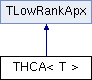HLIBpro  2.9.1
THCA< T > Class Template Reference

uses hybrid cross approximation (HCA) for computing low rank approximation More...

#include <TLowRankApx.hh>

Inheritance diagram for THCA< T >:## Classes

struct  stat_t

class  TGeneratorFn
class defining kernel generator function used by HCA More...

## Public Member Functions

THCA (const coeff_fn_t *coeff, const TGeneratorFn *generator, const real_t aca_eps, const uint ipol_order)

virtual TMatrixbuild (const TBlockCluster *bc, const TTruncAcc &acc) const

virtual TMatrixbuild (const TBlockIndexSet &, const TTruncAcc &) const

virtual bool has_statistics () const
indicate if algorithm provides statistics

## Protected Member Functions

void setup_interpolation ()
build interpolation points for unit cube in $$R^d$$

void transform_ipol_points (const TGeomCluster *cl, tensor_grid_t &grid, const uint ipol_order) const
transform interpolation points to local cluster

void compute_row (const idx_t row_idx, const tensor_grid_t &rowcl_grid, const tensor_grid_t &colcl_grid, const BLAS::Matrix< value_t > &A, const BLAS::Matrix< value_t > &B, const size_t rank, BLAS::Vector< value_t > &row, const uint ipol_order) const
compute single corrected row of kernel generator matrix

void compute_col (const idx_t col_idx, const tensor_grid_t &rowcl_grid, const tensor_grid_t &colcl_grid, const BLAS::Matrix< value_t > &A, const BLAS::Matrix< value_t > &B, const size_t rank, BLAS::Vector< value_t > &col, const uint ipol_order) const
compute single corrected row of kernel generator matrix

void compute_U (const TIndexSet &rowis, const size_t rank, BLAS::Matrix< value_t > &U, const std::vector< idx_t > &col_pivot, const tensor_grid_t &colcl_grid, const uint ipol_order) const
compute matrix U

void compute_V (const TIndexSet &colis, const size_t rank, BLAS::Matrix< value_t > &V, const std::vector< idx_t > &row_pivot, const tensor_grid_t &rowcl_grid, const uint ipol_order) const
compute matrix V

## Detailed Description

### template<typename T> class HLIB::THCA< T >

     THCA provides a low rank approximation algorithm with a guaranteed
approximation quality. It is based on the <em>generator function</em> \f$\gamma(x,y)\f$
of a BEM kernel function \f$k(x,y)\f$ and it's derivatives \f$D_x \gamma(x, y_{l}) \f$
and \f$D_y \gamma(x, y_{l}) \f$.

The class THCA needs a user implemented generator function of type TGeneratorFn, in which
the function itself and the integrals of the corresponding derivates are defined.

Furthermore, HCA is based on interpolation, of which the order defines the accuracy of
the final result. This interpolation order together with an accuracy for the approximation
of the generator function is specific to the given problem, and hence, user defined.


## ◆ THCA()

 THCA ( const coeff_fn_t * coeff, const TGeneratorFn * generator, const real_t aca_eps, const uint ipol_order )

construct HCA based on matrix coefficient function coeff and kernel generator function generator.

The accuracy of the HCA algorithm is defined by aca_eps, the relative accuracy of the internal ACA algorithm and the interpolation order ipol_order.

## ◆ build() [1/2]

 virtual TMatrix* build ( const TBlockCluster * bc, const TTruncAcc & acc ) const
virtual

build low rank matrix for block cluster bct with rank defined by accuracy acc

Reimplemented from TLowRankApx.

## ◆ build() [2/2]

 virtual TMatrix* build ( const TBlockIndexSet & bis, const TTruncAcc & acc ) const
inlinevirtual

build low rank matrix for block index set bis with rank defined by accuracy acc

Implements TLowRankApx.